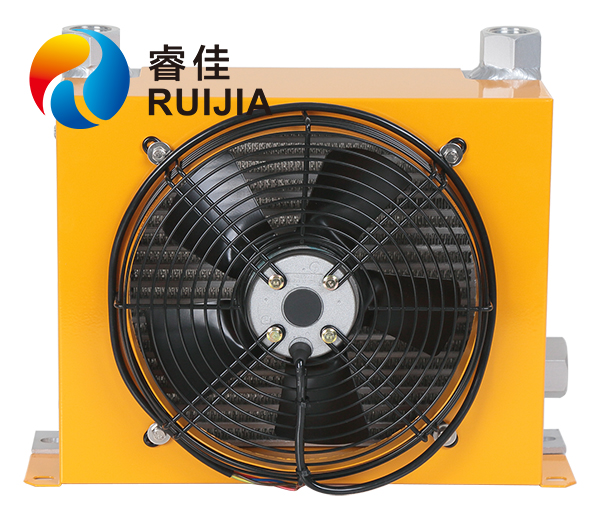﻿ 行业知识：液压冷却器冷却速率

行业知识：液压冷却器冷却速率对 dT/dt=-k(T-C) 进行积分,得
ln(T-C)=-kt+B(B为积分常数)
(T-C)=e^(-kt+B) (1)
设t=0,也就是物体的初温,(1)变成
(T0-C)=e^B
然后代入 (1) 得
T=C+(T0-C)^(-kt)

您的浏览历史